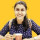1. Home >
2. Apps >
3. Groups >

# GATE Electronics & Communication Engineering Sample Questions - II

Question asked by Ankita Katdare in #Electronics on Jul 8, 2011Ankita Katdare · Jul 8, 2011
Rank A1 - PRO
1 A digital-to-analog converter with a full-scale output voltage of 3.5 V has a resolution close to 14m V. Its bit size is
A) 4
B) 8
C) 16
D) 32

2 A single-phase half-controlled rectifier is driving a separately excited dc motor. The dc motor has a back emf constant of 0.5 V/rpm. The armature current is 5 A without any ripple. The armature resistance is 2W. The converter is working from a 280 V, single phase ac source with a firing angle of 80°. Under this operating condition, the speed of the motor will be
A) 339 rpm
B) 359 rpm
C) 366 rpm
D) 386 rpm

3 In relation to the synchronous machines, which one of the following statements is false?
A) In salient pole machines, the direct-axis synchronous reactance is greater than the quadrature-axis synchronous reactance
B) The damper bars help the synchronous motor self start
C) Short circuit ratio is the ratio of the field current required to produce the rated voltage on open circuit to the rated armature current
D) The V-curve of a synchronous motor represents the variation in the armature current with field excitation, at a given output power

4 A parallel plate air-filled capacitor has plate area of l0-4 m2 and plate separation of 10-3 m. It is connected to a 0.5 V, 3.6 GHz source. The magnitude of the displacement current is (e0 = 1/36p x 10-9 F/m)
A) 10 mA
B) 100 mA
C) 10 A
D) 1.59 mA

5 The 8085 assembly language instruction that stores the content of H and L registers into the memory locations 2050H and 2051H, respectively, is
A) SPHL 2050H
B) SPHL2051H
C) SHLD 2050H
D) STAX 2050H

6 If E is the electric field intensity, Ñ(Ñ x E ) is equal to
A) E
B) | E |
C) null vector
D) zero

7 The insulation strength of an EHV transmission line is mainly governed by
B) switching over-voltages
C) harmonics
D) corona

8 The Q - meter works on the principle of
A) mutual inductance
B) self inductance
C) series resonance
D) parallel resonance

9 A 800 kV transmission line is having per phase line inductance of 1.1 mH/km and per phase line capacitance of 11.68 nF/km. Ignoring the length of the line, its ideal power transfer capability in MW is
A) 1204 MW
B) 1504 MW
C) 2085 MW
D) 2606 MW

10 In a PCM system, if the code word length is increased from 6 to 8 bits, the signal to quantization noise ratio improves by the factor
A) 8/6
B) 12
C) 16
D) 8

11 At an industrial sub-station with a 4 MW load, a capacitor of 2 MVAR is installed to maintain the load power factor at 0.97 lagging. If the capacitor goes out of serivce, the load power factor becomes
A) 0.85
B) 1.00
C) 0.80 lag
D) 0.90 lag

12 The conduction loss versus device current characteristic of a power MOSFET is best approximated by
A) a parabola
B) a straight line
C) a rectangular hyperbola
D) an exponentially decaying function

13 High Voltage DC (HVDC) transmission is mainly used for
A) bulk power transmission over very long distances
B) inter-connecting two systems with the same nominal frequency
C) eliminating reactive power requirement in the operation
D) minimizing harmonics at the converter stations

14 For the equation,
s3 - 4s2+ s + 6 = 0
the number of roots in the left half of s-plane will be

A) 0
B) 1
C) 2
D) 3